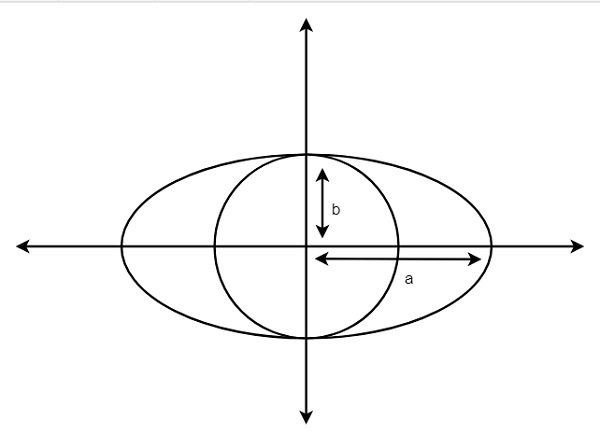# Find the area of largest circle inscribed in ellipse in C++

Suppose we have an ellipse, with major and minor axis length 2a & 2b. We have to find the area of the largest circle that can be inscribed in it. So if the a = 5 and b = 3, then area will be 28.2734From the we can see that the radius of the maximum area circle inscribed in an ellipse will be the minor axis ‘b’. So the area will be A = π*b*b

## Example

Live Demo

#include<iostream>
using namespace std;
double inscribedCircleArea(double b) {
double area = 3.1415 * b * b;
return area;
}
int main() {
double a = 10, b = 8;
cout << "Area of the circle: " << inscribedCircleArea(b);
}

## Output

Area of the circle: 201.056